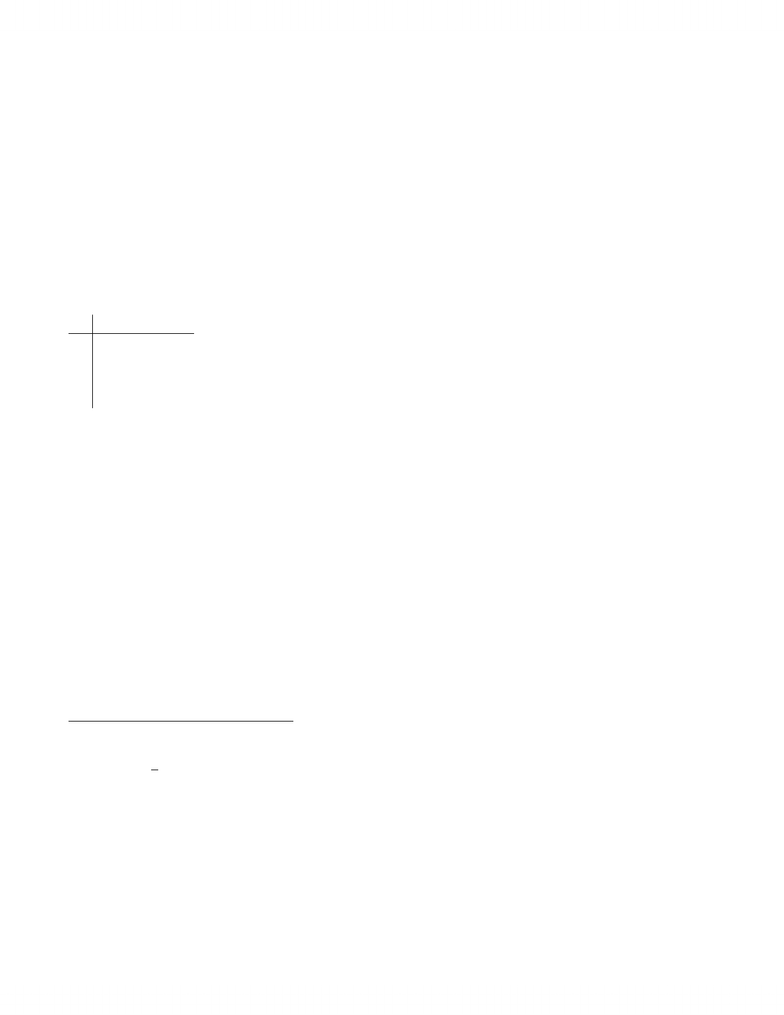Class Notes (1,200,000)
CA (650,000)
UW (20,000)
MATH (2,000)
MATH135 (300)
Lecture 2

# Lecture 2 Notes Winter 2009 Lecture 2 notes

This preview shows page 1. to view the full 4 pages of the document.MATH 135 Winter 2009
Lecture II Notes
Patterns and Conjectures
We often use patterns in mathematics to guess what is happening in general (that is, make a “con-
jecture”), and then try to prove our pattern/conjecture (that is, turn it into a theorem).
Consider the following problem:
npoints are chosen on the circumference of a circle in such a way that when all pairs of
points are connected, no three of these lines intersect at a single point. How many regions
are formed inside the circle?
Let’s try some values of n:
n# of Regions
1 1
2 2
3 4
4 8
So what does pattern appear to be?
# Regions = 2n1
Check: When n= 5, we get 16 regions. Great!
Of course, we’d need to try to prove that this works. (Or do we need to? Isn’t it obvious?)
What happens when n= 6? We would bet the farm on 32. But we get 31 regions, no matter
what we do. (Proving this fact is a diﬀerent story, since we can’t really try all possible positions for
the sixth line.)
So the pattern fails. This again emphasizes why proof is important. While we were able to come
up with a counterexample relatively quickly, this might not always be the case. Without proving a
statement, how can we be absolutely certain? We can’t be.
This comes back to the precision idea as well – starting with the sequence 1, 2, 4, 8, 16, we need to
give a rule in order to ﬁnd the next term. So proof and precision are very important.
Types of Statements to Prove
There are two basic types of statements that we try to prove in mathematics:
1) Simple statements: A
eg. “2 is irrational”
2) Conditional statements: AB(“Aimplies B” or “If Athen B”)
eg. “If nis an odd integer, then n21 is divisible by 4.”
Here, A= “nis an odd integer” is our hypothesis and B= “n21 is divisible by 4” is our
conclusion. Aand Bare both statements on their own about some unknown number n.
To prove propositions of Type 1, we use the axioms of mathematics, logic, our ingenuity, and other
results we know to be true.
To prove propositions of Type 2, we use the hypothesis (or hypotheses) and the list of tools above.
In doing so, we assume that Ais TRUE and use this to derive B. We do not care here what happens